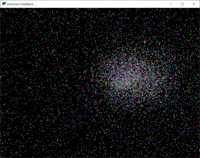# Transform Feedback#transform_feedback.py#
 ``` 1 2 3 4 5 6 7 8 9 10 11 12 13 14 15 16 17 18 19 20 21 22 23 24 25 26 27 28 29 30 31 32 33 34 35 36 37 38 39 40 41 42 43 44 45 46 47 48 49 50 51 52 53 54 55 56 57 58 59 60 61 62 63 64 65 66 67 68 69 70 71 72 73 74 75 76 77 78 79 80 81 82 83 84 85 86 87 88 89 90 91 92 93 94 95 96 97 98 99 100 101 102 103 104 105 106 107 108 109 110 111 112 113 114 115 116 117 118 119 120 121 122 123 124 125 126 127 128 129 130 131 132 133 134 135 136 137 138 139 140 141 142 143 144 145 146 147 148 149 150``` ```""" Shows simple use of transform feedback. Transforming is similar to rendering except that the output or the shader is a buffer instead of a framebuffer/screen. This examples shows a common ping-pong technique were we transform a buffer with positions and velocities between two buffers so we always work on the previous state. * A list of N points are initialized with random positions and velocities * A point of gravity is moving around on the screen affecting the points Using transforms in this way makes us able to process a system that is reacting to external forces in this way. There are no predetermined paths and they system just lives on its own. """ from array import array import math import time import random import arcade from arcade.gl import BufferDescription # Do the math to figure out our screen dimensions SCREEN_WIDTH = 800 SCREEN_HEIGHT = 600 SCREEN_TITLE = "Transform Feedback" class MyGame(arcade.Window): def __init__(self, width, height, title): super().__init__(width, height, title, resizable=True) self.time = 0 # Program to visualize the points self.points_progran = self.ctx.program( vertex_shader=""" #version 330 in vec2 in_pos; out vec3 color; void main() { // Let's just give them a "random" color based on the vertex id color = vec3( mod((gl_VertexID * 100 % 11) / 10.0, 1.0), mod((gl_VertexID * 100 % 27) / 10.0, 1.0), mod((gl_VertexID * 100 % 71) / 10.0, 1.0)); // Pass the point position to primitive assembly gl_Position = vec4(in_pos, 0.0, 1.0); } """, fragment_shader=""" #version 330 // Color passed in from the vertex shader in vec3 color; // The pixel we are writing to in the framebuffer out vec4 fragColor; void main() { // Fill the point fragColor = vec4(color, 1.0); } """, ) # A program transforming points being affected by a gravity point self.gravity_program = self.ctx.program( vertex_shader=""" #version 330 // Delta time (since last frame) uniform float dt; // Strength of gravity uniform float force; // Position of gravity uniform vec2 gravity_pos; // The format of the data in our transform buffer(s) in vec2 in_pos; in vec2 in_vel; // We are writing to a buffer of the same format out vec2 out_pos; out vec2 out_vel; void main() { // Simplified gravity calculations vec2 dir = normalize(gravity_pos - in_pos) * force; vec2 vel = in_vel + dir / length(dir) * 0.01; // Write to the output buffer out_vel = vel; out_pos = in_pos + vel * dt; } """, ) N = 50_000 # Make two buffers we transform between so we can work on the previous result self.buffer_1 = self.ctx.buffer(data=array('f', self.gen_initial_data(N))) self.buffer_2 = self.ctx.buffer(reserve=self.buffer_1.size) # We also need to be able to visualize both versions (draw to the screen) self.vao_1 = self.ctx.geometry([BufferDescription(self.buffer_1, '2f 2x4', ['in_pos'])]) self.vao_2 = self.ctx.geometry([BufferDescription(self.buffer_2, '2f 2x4', ['in_pos'])]) # We need to be able to transform both buffers (ping-pong) self.gravity_1 = self.ctx.geometry([BufferDescription(self.buffer_1, '2f 2f', ['in_pos', 'in_vel'])]) self.gravity_2 = self.ctx.geometry([BufferDescription(self.buffer_2, '2f 2f', ['in_pos', 'in_vel'])]) self.ctx.enable_only() # Ensure no context flags are set self.time = time.time() def gen_initial_data(self, count): for _ in range(count): yield random.uniform(-1.2, 1.2) # pos x yield random.uniform(-1.2, 1.2) # pos y yield random.uniform(-.3, .3) # velocity x yield random.uniform(-.3, .3) # velocity y def on_draw(self): self.clear() self.ctx.point_size = 2 * self.get_pixel_ratio() # Calculate the actual delta time and current time t = time.time() frame_time = t - self.time self.time = t # Set uniforms in the program self.gravity_program['dt'] = frame_time self.gravity_program['force'] = 0.25 self.gravity_program['gravity_pos'] = math.sin(self.time * 0.77) * 0.25, math.cos(self.time) * 0.25 # Transform data in buffer_1 into buffer_2 self.gravity_1.transform(self.gravity_program, self.buffer_2) # Render the result (Draw buffer_2) self.vao_2.render(self.points_progran, mode=self.ctx.POINTS) # Swap around stuff around so we transform back and fourth between the two buffers self.gravity_1, self.gravity_2 = self.gravity_2, self.gravity_1 self.vao_1, self.vao_2 = self.vao_2, self.vao_1 self.buffer_1, self.buffer_2 = self.buffer_2, self.buffer_1 if __name__ == "__main__": window = MyGame(SCREEN_WIDTH, SCREEN_HEIGHT, SCREEN_TITLE) window.center_window() arcade.run() ```